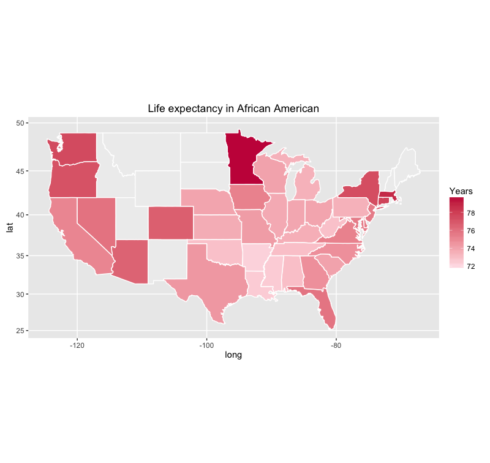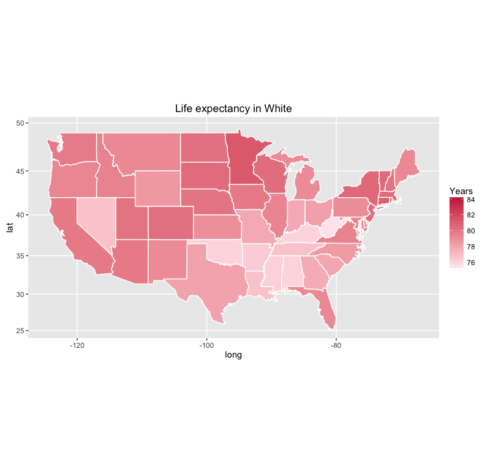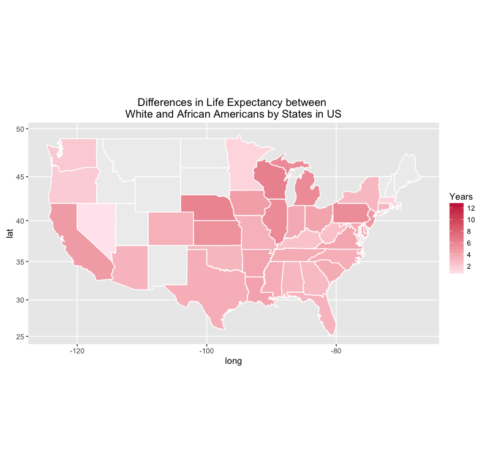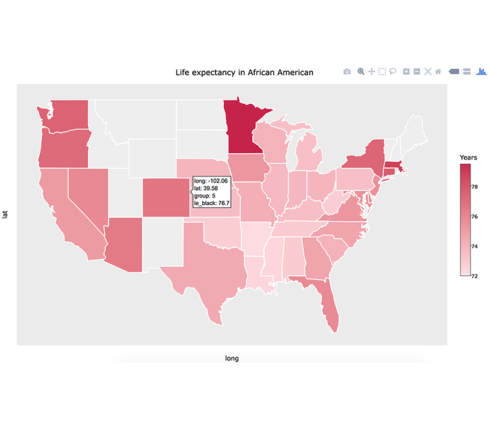#.

Original post is published at DataScience+

Recently, I become interested to grasp the data from webpages, such as Wikipedia, and to visualize it with R. As I did in my previous post, I use `rvest` package to get the data from webpage and `ggplot` package to visualize the data.
In this post, I will map the life expectancy in White and African-American in US.
` ## LOAD THE PACKAGES #### library(rvest) library(ggplot2) library(dplyr) library(scales)`

Import the data from Wikipedia.
` ## LOAD THE DATA #### le = read_html("https://en.wikipedia.org/wiki/List_of_U.S._states_by_life_expectancy") le = le %>% html_nodes("table") %>% .[]%>% html_table(fill=T)`
Now I have to clean the data. Below I have explain the role of each code.
` ## CLEAN THE DATA #### # select only columns with data le = le[c(1:8)] # get the names from 3rd row and add to columns names(le) = le[3,] # delete rows and columns which I am not interested le = le[-c(1:3), ] le = le[, -c(5:7)] # rename the names of 4th and 5th column names(le)[c(4,5)] = c("le_black", "le_white") # make variables as numeric le = le %>% mutate( le_black = as.numeric(le_black), le_white = as.numeric(le_white))`

Since there are some differences in life expectancy between White and African-American, I will calculate the differences and will map it.
` le = le %>% mutate(le_diff = (le_white - le_black))`

I will load the map data and will merge the datasets togather.
`## LOAD THE MAP DATA #### states = map_data("state") # create a new variable name for state le\$region = tolower(le\$State) # merge the datasets states = merge(states, le, by="region", all.x=T)`

Now its time to make the plot. First I will plot the life expectancy in African-American in US. For few states we don't have the data, and therefore I will color it in grey color.
`## MAKE THE PLOT #### # Life expectancy in African American ggplot(states, aes(x = long, y = lat, group = group, fill = le_black)) + geom_polygon(color = "white") + scale_fill_gradient(name = "Years", low = "#ffe8ee", high = "#c81f49", guide = "colorbar", na.value="#eeeeee", breaks = pretty_breaks(n = 5)) + labs(title="Life expectancy in African American") + coord_map()`
Here is the plot:The code below is for White people in US.
` # Life expectancy in White American ggplot(states, aes(x = long, y = lat, group = group, fill = le_white)) + geom_polygon(color = "white") + scale_fill_gradient(name = "Years", low = "#ffe8ee", high = "#c81f49", guide = "colorbar", na.value="Gray", breaks = pretty_breaks(n = 5)) + labs(title="Life expectancy in White") + coord_map()`
Here is the plot:Finally, I will map the differences between white and African American people in US.
` # Differences in Life expectancy between White and African American ggplot(states, aes(x = long, y = lat, group = group, fill = le_diff)) + geom_polygon(color = "white") + scale_fill_gradient(name = "Years", low = "#ffe8ee", high = "#c81f49", guide = "colorbar", na.value="#eeeeee", breaks = pretty_breaks(n = 5)) + labs(title="Differences in Life Expectancy between \nWhite and African Americans by States in US") + coord_map()`
Here is the plot:On my previous post I got a comment to add the pop-up effect as I hover over the states. This is a simple task as Andrea exmplained in his comment. What you have to do is to install the `plotly` package, to create a object for ggplot, and then to use this function `ggplotly(map_plot)` to plot it.
` library(plotly) map_plot = ggplot(states, aes(x = long, y = lat, group = group, fill = le_black)) + geom_polygon(color = "white") + scale_fill_gradient(name = "Years", low = "#ffe8ee", high = "#c81f49", guide = "colorbar", na.value="#eeeeee", breaks = pretty_breaks(n = 5)) + labs(title="Life expectancy in African American") + coord_map() ggplotly(map_plot)`
Here is the plot: